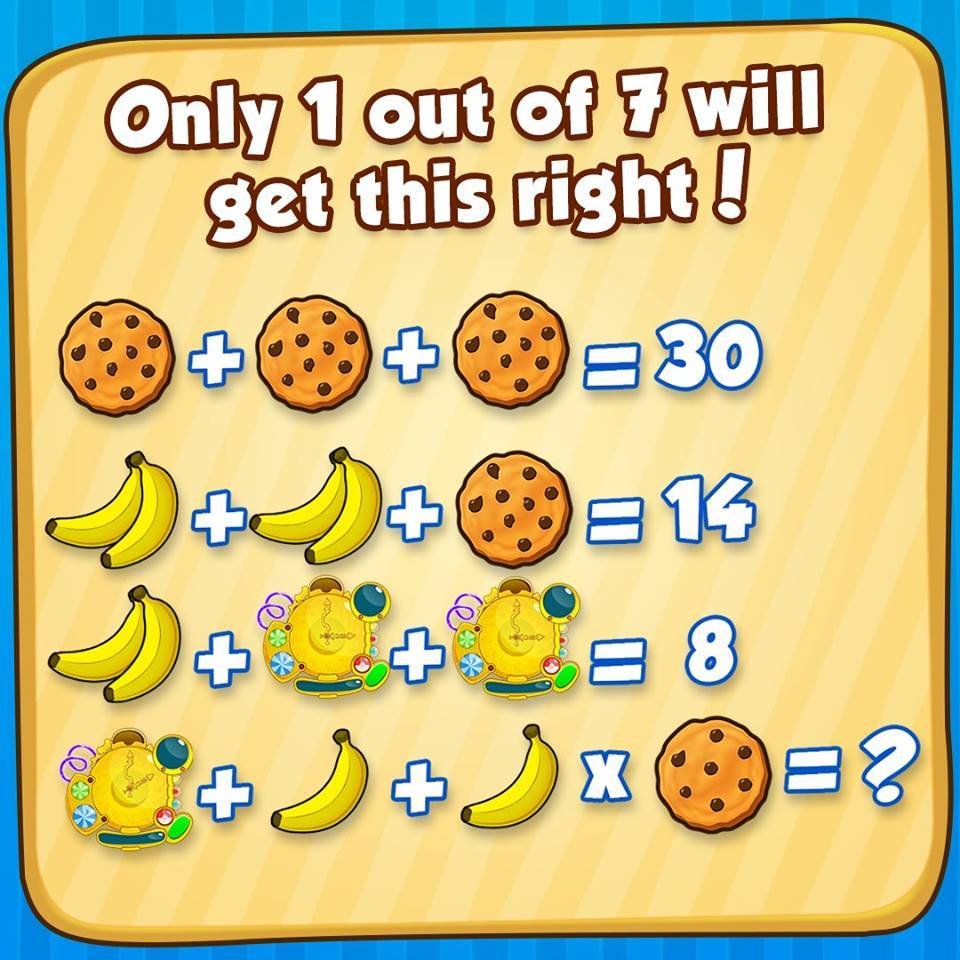Can you solve this Cookie Banana Clock Puzzle? So many people on Social media are going crazy over this puzzle. No-one seems to agree with each others answer. Lets see if you answer it correctly. Here is puzzle for you.Try solving this, once you have an answer then scroll down and match your answer with one we have posted here.

Also Try: Arrow Up to Down Triangle Puzzle

Look closely and notice 2 things in above picture:

1. No. of chocolate pieces on each cookie
2. Time in clock

2 bananas + 2 bananas + Cookie(10 chocs)  = 14

4 Bananas + 10 =14 ==>  Banana = 1

2 Bananas + Clock(3 O’clock) + Clock(3 O’clock)  = 8

2*1 + 2 Clock(3 O’clock)  = 8 ==> Clock(3 O’clock) = 3

Clock(2 O’clock) + banana + banana X Cookie with 7 chocs = ??

Now remember the PEDMAS rule.

Here’s an explanation of the rules given in PEMDAS:

1. P as the first letter means you complete any calculations in grouping symbols first.
2. Next, look for exponents, E. Ignore any other operation, and take any numbers with exponents to their respective powers.
3. Even though M for multiplication in PEMDAS comes before D for division, these two operations actually have the same priority. Complete only those two operations in the order they occur from left to right.
4. Even though A for addition is in PEMDAS before S for subtraction, these two operations also have the same priority. You look for these last two operations from left to right and complete them in that order.

We have already resolved values of each item which is:

Cookie with 10 chocs = 10 ==> it means each choc piece is equal to 1

Banana = 1

Clock with time as 3’o clock = 3 ==> it means if clock is at 1 o’clock value would be 1

So as per equation values would be:

Equation: Clock(2 O’clock) + banana + banana X Cookie with 7 chocs = ??

2 + 1 + 1 X 7 ==> 2 + 1 + 7 = 10

Answer of above puzzle is 10

Please use comment section if you think answer is wrong. Also, don’t forget to follow us on Facebook. We daily publish new puzzles, riddles and brainteasers on our Facebook page.

## 8 Replies to “Cookie Banana Clock Puzzle”

1.Daryll Kelly says:

My business partner was married to a guy with a PhD in mathematics and managed to accrue assets if about \$2 Million in 30 years. I am a high school dropout and our partnership increased her net worth by \$4 million in 3 years. Using the logic applied to your puzzle I am pleased that I am not handicapped
by education. There are no brackets in the puzzle quarantining any particular action, so therefore one should proceed in logical order from left to right

2.Dawn Duncan says:

I didn’t even go to high school, and even I know that when the parentheses aren’t physically in the calculation, one is to mentally insert them where they would normally go, which is around the multiplication. One does not, in fact, simply proceed from left to right in “logical” order.

3.Kevin Roscoe says:

I’m a 2 year old and may or may not go to any school but there is nothing in this puzzle that instructs me to count the chocolate chips or concern myself with what time is on the clock face . Which by the way might be 3:00 or 15:00. There for ,the cookies are a value of 10 each. The clock must be a value of 3 and the double banana a value of 2. Assuming that a single banana would be 1/2 or 50% of two bananas, then simple math tells me that it’s time for a glass of MILK!

4.Michelle says:

The real answer is 14. You cannot separate the cookie into 10 chips. If you do, how are you accounting for the cookie itself? The cookie should ve viewed at 1 whoke unit.

5.brdybnch0401 says:

10 is the correct answer. I find it amazing that people will post how intelligent they are but yet never fact check themselves. When in essence they most generally in the end prove their own ignorance. Order of operation or PEMDAS (also has a few other appreviations) says first you do parenthesis, then exponents, then multiplication/division, then addition/subtraction. In this particular picture the clock changed from 3 o’clock to 2 o’clock. The Banana changed from having 2 to having 1. The cookie changed from 10 chips to having 7 chips. Therefore your problem is 2+1+1×7. Keeping mind the order of operation you first multiply 1×7=7. This now leaves you with 2+1+7 which equals 10.

6.prashant says:

7.Rich Corbett 🇺🇸 (@RichC) says:

The answer 10 is only “half correct” … that is for when it is in the AM.
If it were PM using military time (24 hr clock), there would be a second answer of 22.

8.Stacy says:

The answer is 28. There are no grouping symbols in the equation. You can’t assume facts that are not in evidence.

9.Laura says:

The chips are the facts

So I see problem as..
2+1+(1×7)= 10

You see as…
(2+1+1)x7=28